# Electrical Force AP Physics B Electric Charge Charge

• Slides: 21Electrical Force AP Physics BElectric Charge “Charge” is a property of subatomic particles. Facts about charge: • There are 2 types basically, positive (protons) and negative (electrons) • LIKE charges REPEL and OPPOSITE charges ATTRACT • Similar to mass; exert forces. – Difference: there is no negative mass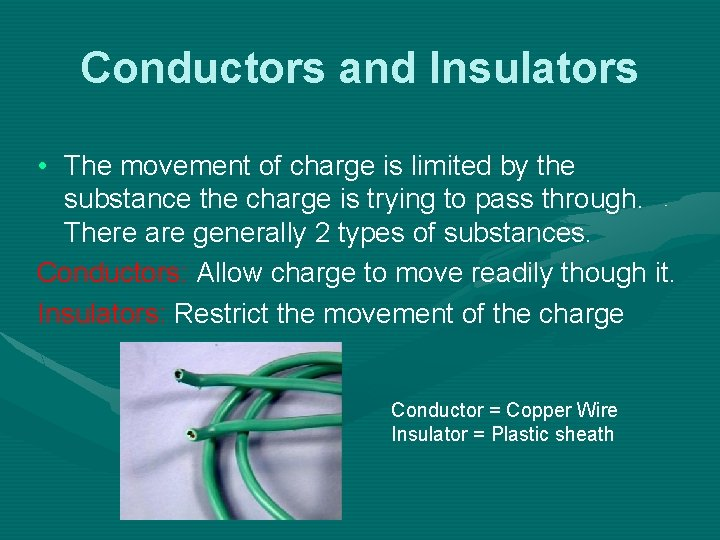Conductors and Insulators • The movement of charge is limited by the substance the charge is trying to pass through. There are generally 2 types of substances. Conductors: Allow charge to move readily though it. Insulators: Restrict the movement of the charge Conductor = Copper Wire Insulator = Plastic sheathElectric Charge – The specifics Some important constants: Particle Proton • The symbol for CHARGE is “q” • The unit is the COULOMB (C), named after Charles Coulomb • If we are talking about a SINGLE charged particle such as 1 electron or 1 proton we are referring to an ELEMENTARY charge and often use, e , to symbolize this. Charge + 1. 6 x 10 -19 C - 1. 6 x 10 -19 C Mass 1. 67 x 10 -27 kg Electron 9. 11 x 10 -31 kg These are given constants. Neutron 0 1. 67 x 10 -27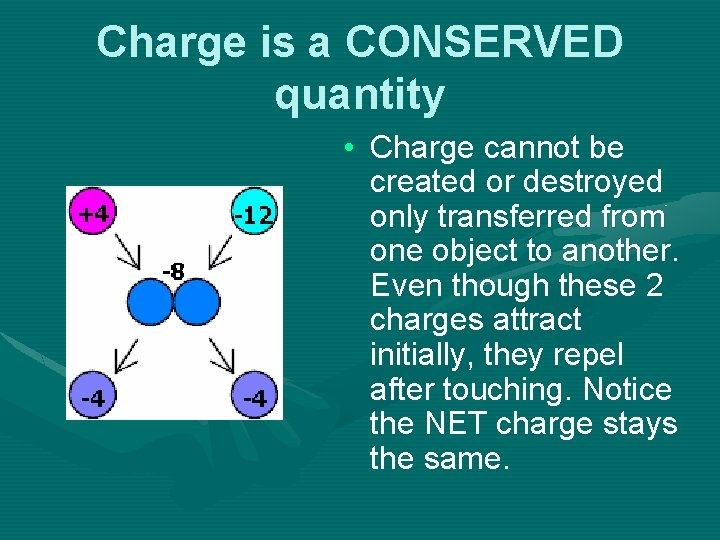Charge is a CONSERVED quantity • Charge cannot be created or destroyed only transferred from one object to another. Even though these 2 charges attract initially, they repel after touching. Notice the NET charge stays the same.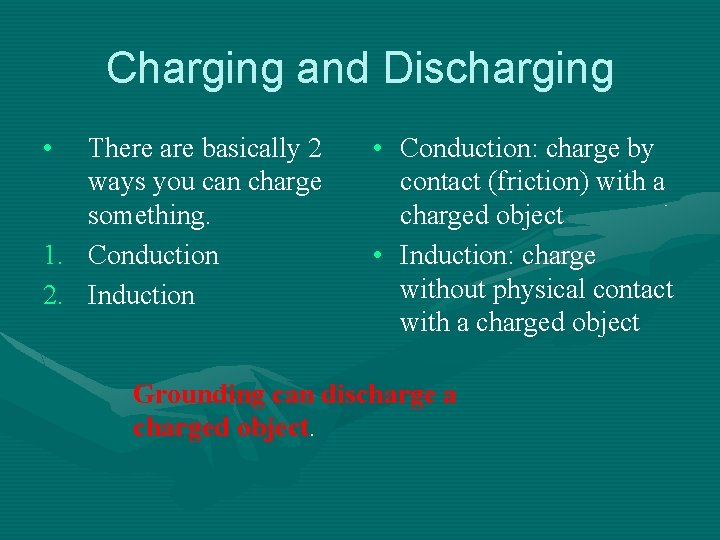Charging and Discharging • There are basically 2 ways you can charge something. 1. Conduction 2. Induction • Conduction: charge by contact (friction) with a charged object • Induction: charge without physical contact with a charged object Grounding can discharge a charged object.Conduction • Ex: charging a neutral metal sphere with a conduction rod.Induction and Grounding • The second way to charge something is via INDUCTION, which requires NO PHYSICAL CONTACT. We bring a negatively charged rod near a neutral sphere. The protons in the sphere localize near the rod, while the electrons are repelled to the other side of the sphere. A wire can then be brought in contact with the negative side and allowed to touch the GROUND. The electrons will always move towards a more massive objects to increase separation from other electrons, leaving a NET positive sphere behind.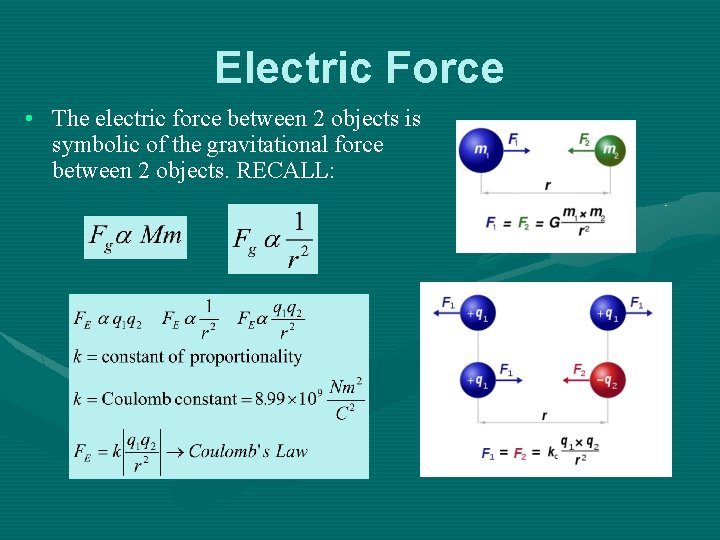Electric Force • The electric force between 2 objects is symbolic of the gravitational force between 2 objects. RECALL: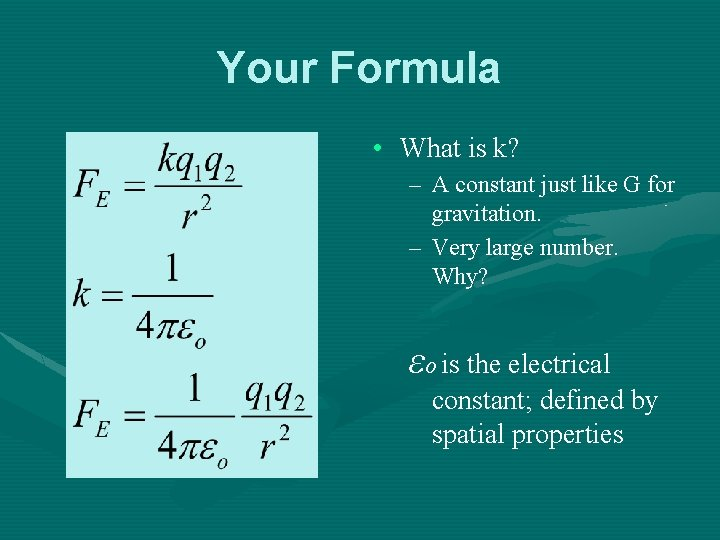Your Formula • What is k? – A constant just like G for gravitation. – Very large number. Why? εo is the electrical constant; defined by spatial properties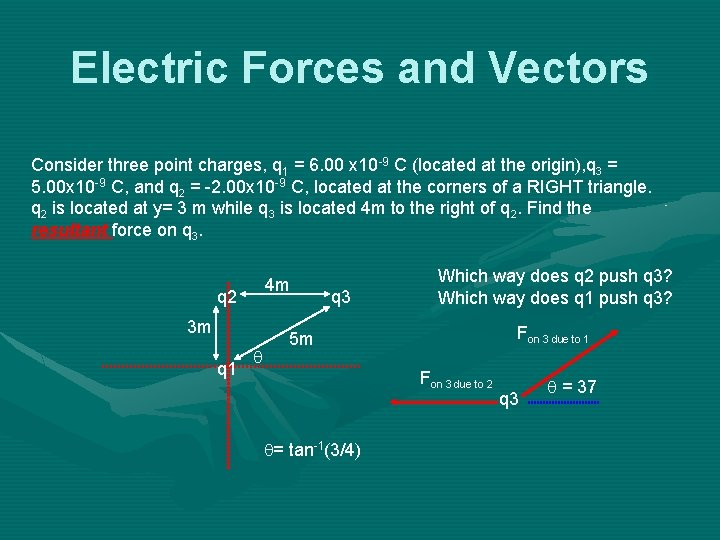Electric Forces and Vectors Consider three point charges, q 1 = 6. 00 x 10 -9 C (located at the origin), q 3 = 5. 00 x 10 -9 C, and q 2 = -2. 00 x 10 -9 C, located at the corners of a RIGHT triangle. q 2 is located at y= 3 m while q 3 is located 4 m to the right of q 2. Find the resultant force on q 3. 4 m q 2 3 m q 1 q q 3 Which way does q 2 push q 3? Which way does q 1 push q 3? Fon 3 due to 1 5 m Fon 3 due to 2 q= tan-1(3/4) q 3 q = 37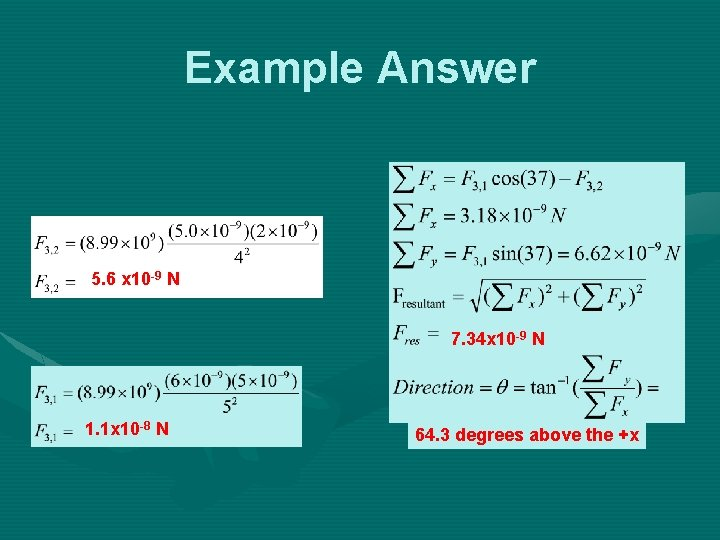Example Answer 5. 6 x 10 -9 N 7. 34 x 10 -9 N 1. 1 x 10 -8 N 64. 3 degrees above the +x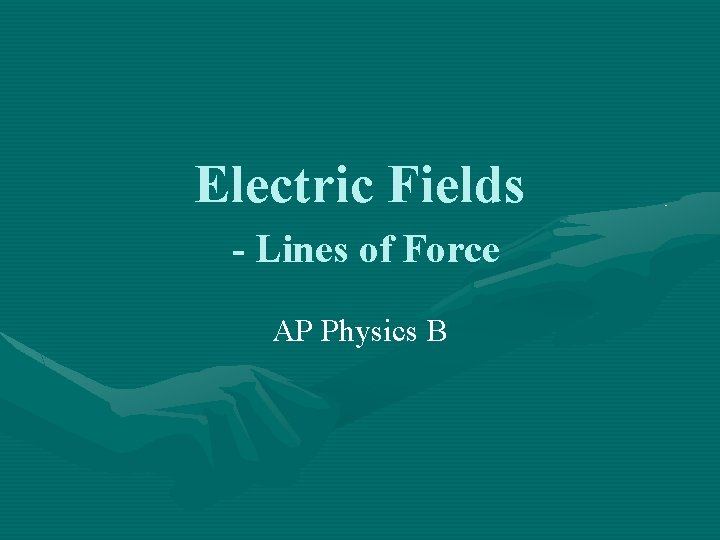Electric Fields - Lines of Force AP Physics BElectric Fields • By definition, they are “LINES OF FORCE” Some important facts: • An electric field is a vector • Always is in the direction that a POSITIVE “test” charge would move • The amount of force PER “test” charge • Away from the positive charge and towards the negative charge. If you placed a 2 nd positive charge (test charge), near the positive charge shown above, it would move AWAY. If you placed that same charge near the negative charge shown above it would move TOWARDS.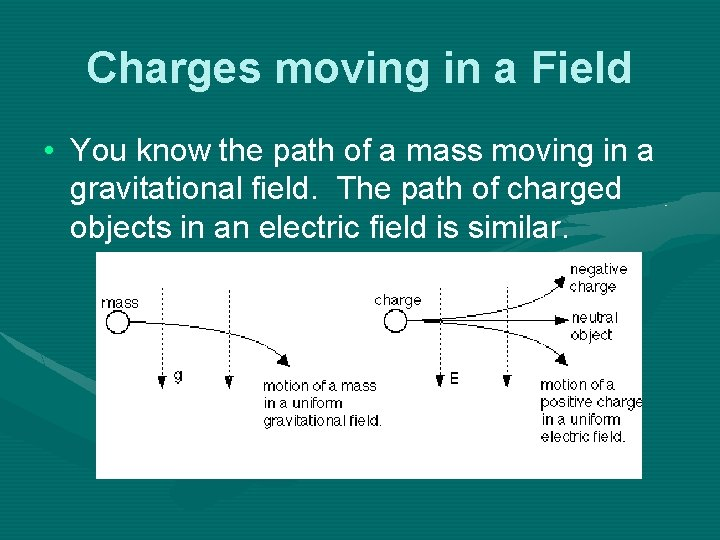Charges moving in a Field • You know the path of a mass moving in a gravitational field. The path of charged objects in an electric field is similar.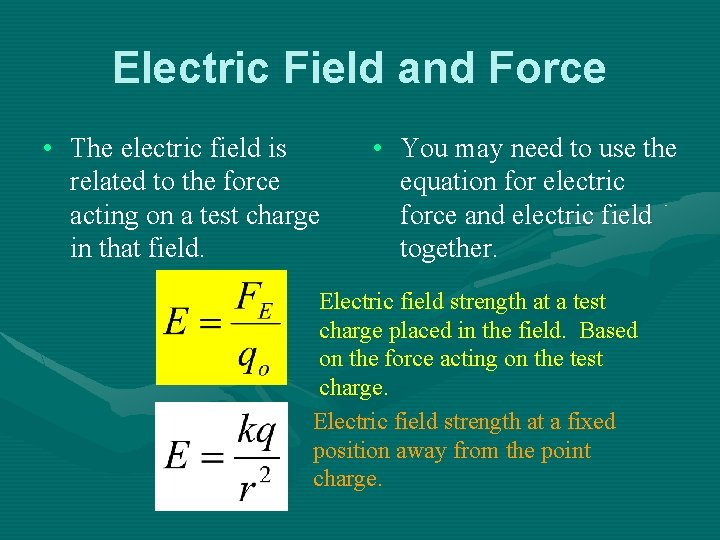Electric Field and Force • The electric field is related to the force acting on a test charge in that field. • You may need to use the equation for electric force and electric field together. Electric field strength at a test charge placed in the field. Based on the force acting on the test charge. Electric field strength at a fixed position away from the point charge.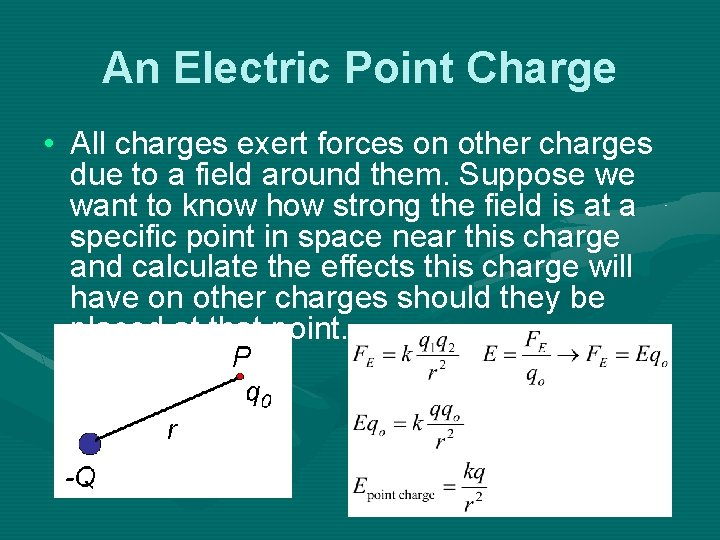An Electric Point Charge • All charges exert forces on other charges due to a field around them. Suppose we want to know how strong the field is at a specific point in space near this charge and calculate the effects this charge will have on other charges should they be placed at that point.Electric Fields and Newton’s Laws • The equation for ELECTRIC FIELD is symbolic of the equation for WEIGHT just like Coulomb’s law is symbolic of Newton’s Law of Gravitation. • The symbol for Electric Field is, “E”. And since it is defined as a force per unit charge the unit is Newton per Coulomb, N/C. • NOTE: the equations above will ONLY help you determine the MAGNITUDE of the field or force. Conceptual understanding will help you determine the direction.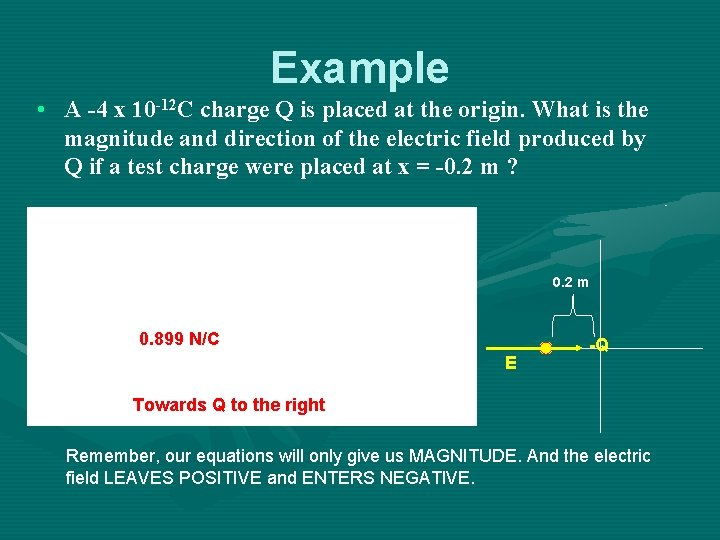Example • A -4 x 10 -12 C charge Q is placed at the origin. What is the magnitude and direction of the electric field produced by Q if a test charge were placed at x = -0. 2 m ? 0. 2 m 0. 899 N/C E -Q Towards Q to the right Remember, our equations will only give us MAGNITUDE. And the electric field LEAVES POSITIVE and ENTERS NEGATIVE.Electric Field of a Conductor • A few more things about electric fields; suppose you bring a conductor NEAR a charged object. The side closest to the charged object will be INDUCED with the opposite charge. However, the charge will ONLY exist on the surface. There will never be an electric field inside a conductor. Insulators, however, can store the charge inside. There must be a positive charge on this side There must be a negative charge on this side